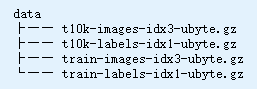# ConnectionResetError: [Errno 104] Connection reset by peer

``````from sklearn.datasets import fetch_mldata
mnist = fetch_mldata('MNIST original')
``````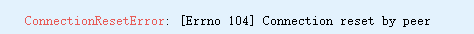solution

``````!wget https://github.com/amplab/datascience-sp14/raw/master/lab7/mldata/mnist-original.mat
``````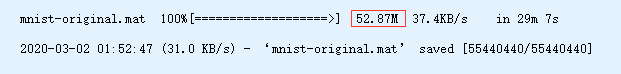2. View the SkLearn data root directory

``````from sklearn.datasets.base import get_data_home
print (get_data_home())
``````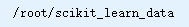``````!tree /root/scikit_learn_data
``````3. Copy the data set to `mldata` directory

``````!cp mnist-original.mat /root/scikit_learn_data/mldata
!tree /root/scikit_learn_data
``````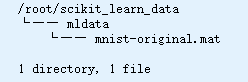4. Verify

``````from sklearn.datasets import fetch_mldata
mnist = fetch_mldata('MNIST original')

mnist
``````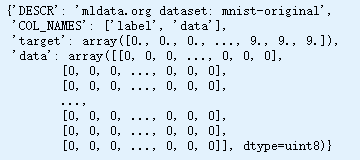5. OK!!!

``````mnist.data.shape
``````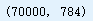``````mnist.target.shape
``````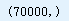``````from sklearn.datasets import fetch_openml
mnist = fetch_openml('mnist_784', version=1)
``````

``````from tensorflow.examples.tutorials.mnist import input_data